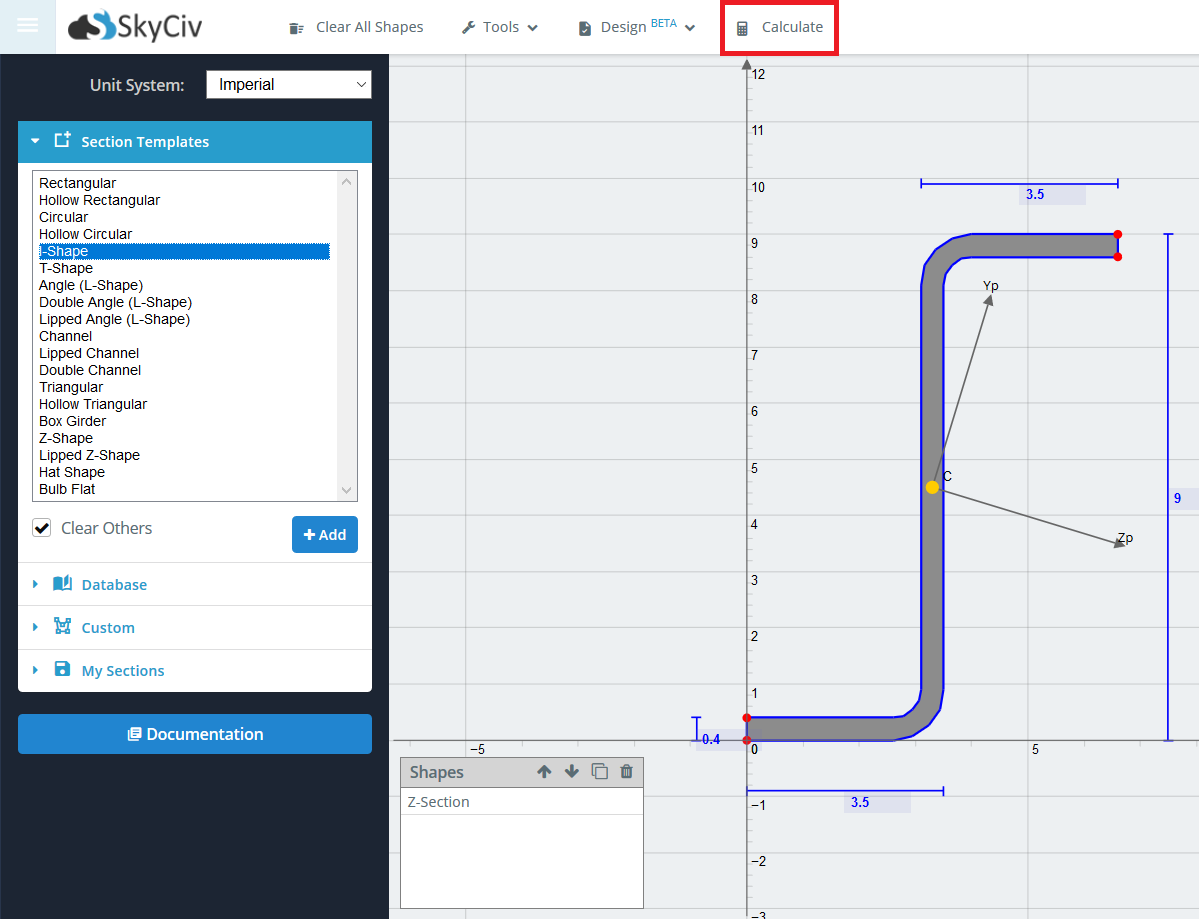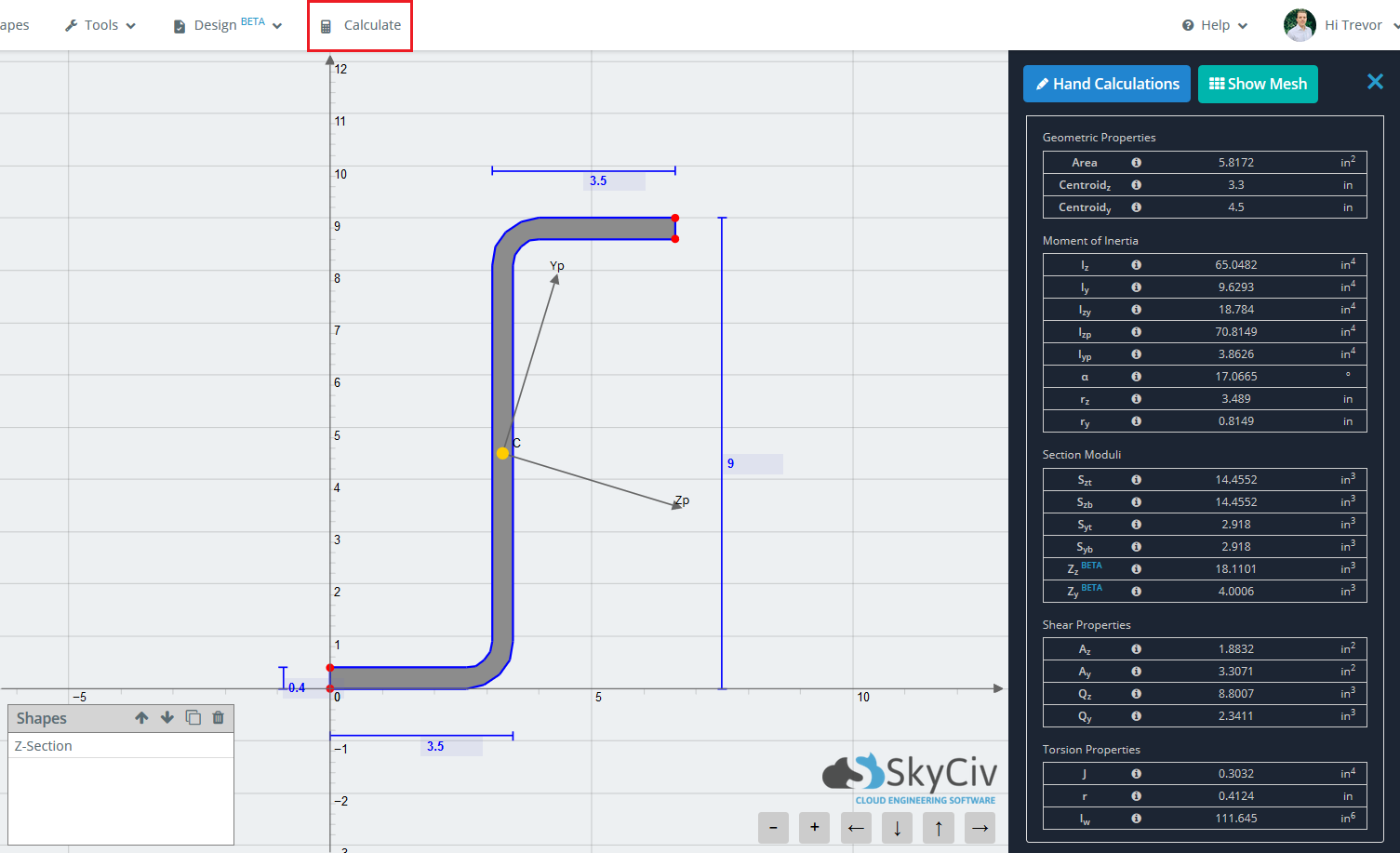Documentazione SkyCiv

La tua guida al software SkyCiv - tutorial, guide pratiche e articoli tecnici

1. Casa
2. SkyCiv Section Builder
3. risultati
4. Risultati standard

# Risultati standard

The main purpose of the Section Builder is to calculate the various section properties of your section. The standard results refer to the results of a section when ignoring material property differences.

When you’ve finished building your section, simply click ‘Calculateon the top toolbar to determine the section properties. Note that the short wait time associated with each calculate is due to the Finite Element Analysis and Meshing that occurs in the background in order to solve for torsion constant, torsion radius, and warping constant. By using a FEA method to calculate these properties, a higher accuracy is achieved. Less robust programs only use approximate equations on the standard sections for these properties and fail to solve when a non standard section (such as the cross beam below) is entered.The results are generally broken down into 5 main categories.

• Proprietà geometriche
• La zona
• centroid (CON)
• centroid (E)
• Moment of Inertia Properties
• A partire dal – Moment of Inertia about the Z-axis
• Iy – Moment of Inertia about the Y-axis
• Izy – Momento d'inerzia
• IzpMoment of Inertia about the principal Z-axis
• IypMoment of Inertia about the principal Y-axis
• un' – angle of rotation of principal axis
• rz – Radius of Gyration about the Z-axis
• ryRadius of Gyration about the Y-axis
• Sezione Moduli
• SztElastic Section Modulus about the Z-axis to the top of the section
• SzbElastic Section Modulus about the Z-axis to the bottom of the section
• SytElastic Section Modulus about the Y-axis to the top of the section
• SybElastic Section Modulus about the Y-axis to the bottom of the section
• ZzPlastic Section Modulus about the Z-axis
• ZyPlastic Section Modulus about the Y-axis
• Proprietà di taglio
• AzShear Area about Z axis
• Ay – Shear Area about Y axis
• Qz – Static Moment of Area about the Z neutral axis
• Qy – Static Moment of Area about the Y neutral axis
• Torsion Properties
• J – Costante di torsione
• r – Raggio di torsione
• e – Costante di deformazioneQuesto articolo ti è stato utile?